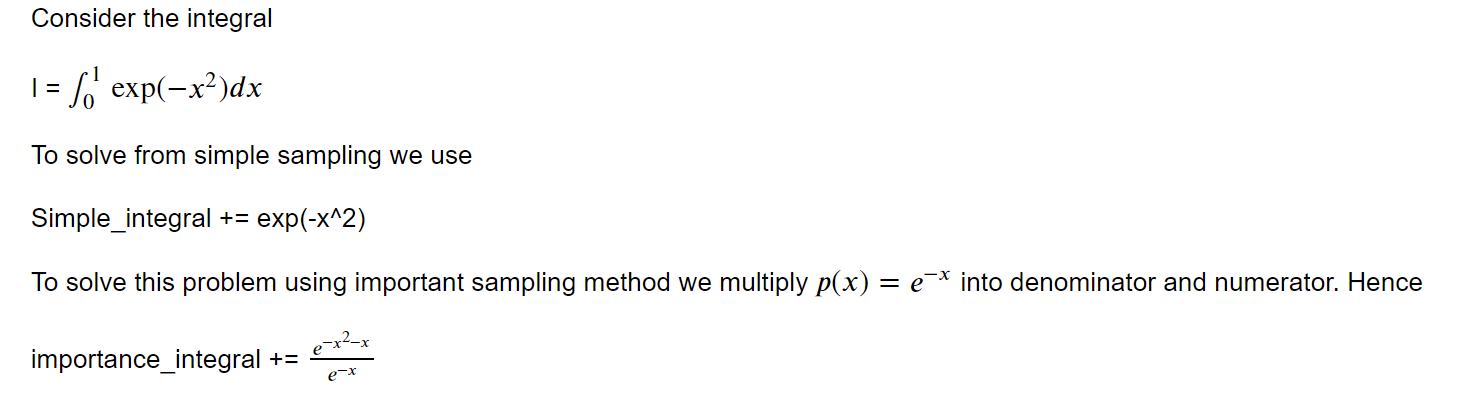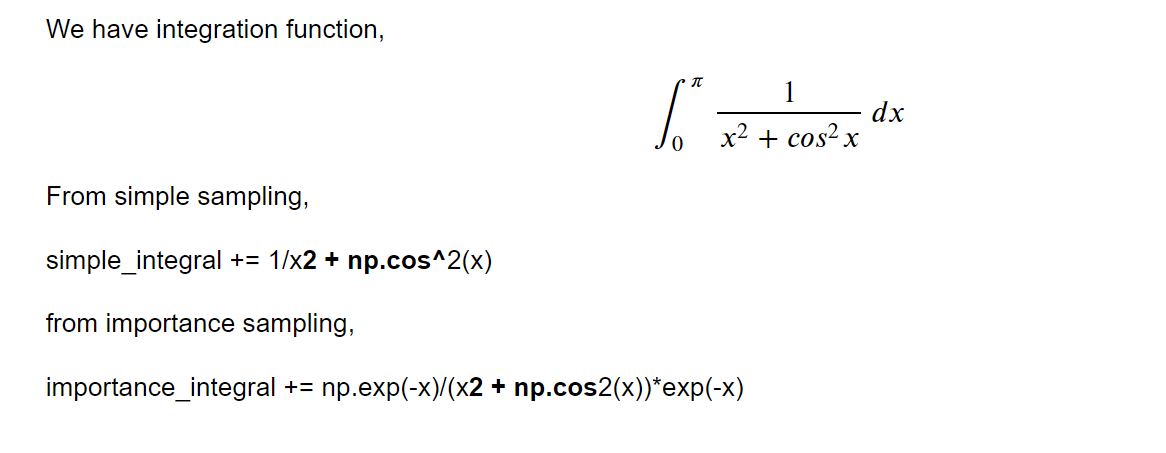We shared the blog, which all are related to Monte Carlo based on simple sampling. To see the previous blogs of Monte Carlo, you can visit the following link.

# Why we used importance sampling instead of simple sampling

In theory, simple sampling techniques can be used to run MC integrations and other simulations. Unfortunately, the majority of the samples generated in this way will only make up a small portion of the equilibrium (time-independent) averages, necessitating the use of more advanced techniques in order to get results that are accurate enough. “Importance Sampling” is one of these techniques.

# Important sampling and simple sampling### Algorithm

1. start
2. Take a number
3. Initialize a variable for simple sampling
4. Initialize a variable for importance sampling
5. Loop with in the range of given number
6. Take a random variable within given range
7. Increment the simple sampling variable by using given function
8. Increment the important sampling variable by using given function
9. Print the result for both
10. Stop
import random
import math
import numpy as np
import matplotlib.pyplot as plt

print("True Mean:")
true_value = np.exp(-1)+ 1
N = [100,1000,10000,100000,100000,1000000]
d = {}
E1 = []
E2 = []
for i in range(len(N)):
integral = 0
importance = 0

for j in range(N[i]):

x = random.random()
integral += np.exp(-x**2)
importance += np.exp(-x**2 - x )/np.exp(-x)
test_value_simple = integral/j
test_value_importance = importance/j
error_simple = abs(test_value_simple - true_value)
E1.append(error_simple)
error_importance = abs(test_value_importance-true_value)
E2.append(error_importance)
print(f"result simple: {test_value_simple}")
print(f"Error from simple:{error_simple}")
print(f"result importance: {test_value_importance}")
print(f"Error from importance:{error_importance}")


True Mean:
result simple: 0.7657479523721085
Error from simple:0.6021314887993339
result importance: 0.7657479523721085
Error from importance:0.6021314887993339
result simple: 0.7335360135333993
Error from simple:0.6343434276380431
result importance: 0.7335360135333993
Error from importance:0.6343434276380431
result simple: 0.7461352168842383
Error from simple:0.621744224287204
result importance: 0.7461352168842383
Error from importance:0.621744224287204
result simple: 0.747056058567544
Error from simple:0.6208233826038984
result importance: 0.7470560585675443
Error from importance:0.620823382603898
result simple: 0.7465081112460847
Error from simple:0.6213713299253576
result importance: 0.7465081112460847
Error from importance:0.6213713299253576
result simple: 0.7464374626347431
Error from simple:0.6214419785366992
result importance: 0.746437462634743
Error from importance:0.6214419785366994


From above result we see that result obtained from simple sampling and importance sampling are approximately equal.

# Important sampling and simple sampling for integration Function### Algorithm

1. start
2. Take a number
3. Initialize a variable for simple sampling
4. Initialize a variable for importance sampling
5. Loop with in the range of given number
6. Take a random variable within given range
7. Increment the simple sampling variable by using given function
8. Increment the important sampling variable by using given function
9. Print the result for both
10. Stop
import scipy as sp


f = lambda x : 1/(x**2 + np.cos(x)**2)

1.5811879708477277

print("True Mean:")
true_value = 1.5811879708477277
N = [100,1000,10000,100000,100000,1000000]
d = {}
E1 = []
E2 = []
for i in range(len(N)):
integral = 0
importance = 0

for j in range(N[i]):

x = random.random()
integral += 1/(x**2 + np.cos(x)**2)
importance += 1*np.exp(-x)/(np.exp(-x)*x**2 + np.exp(-x)*np.cos(x)**2)
test_value_simple = integral/j
test_value_importance = importance/j
error_simple = abs(true_value - test_value_importance)
error_importance = abs(test_value_importance - true_value)
E1.append(error_simple)
E2.append(error_importance)
print(f"Result from Simple: {test_value_simple}")
print(f"Error from Simple:{error_simple}")
print(f"Result from importance: {test_value_importance}")
print(f"Error from importance:{error_importance}")


True Mean:
Result from Simple: 0.9567678930514265
Error from Simple:0.6244200777963012
Result from importance: 0.9567678930514265
Error from importance:0.6244200777963012
Result from Simple: 0.9490487042385376
Error from Simple:0.6321392666091901
Result from importance: 0.9490487042385376
Error from importance:0.6321392666091901
Result from Simple: 0.9472548097390786
Error from Simple:0.6339331611086491
Result from importance: 0.9472548097390786
Error from importance:0.6339331611086491
Result from Simple: 0.9476834937239044
Error from Simple:0.6335044771238233
Result from importance: 0.9476834937239044
Error from importance:0.6335044771238233
Result from Simple: 0.947734842700603
Error from Simple:0.6334531281471246
Result from importance: 0.947734842700603
Error from importance:0.6334531281471246
Result from Simple: 0.9476064786946827
Error from Simple:0.6335814921530448
Result from importance: 0.9476064786946828
Error from importance:0.6335814921530448


We can observe from the above result that the results from simple sampling and importance sampling are roughly equivalent.

Tags:

Categories:

Updated: